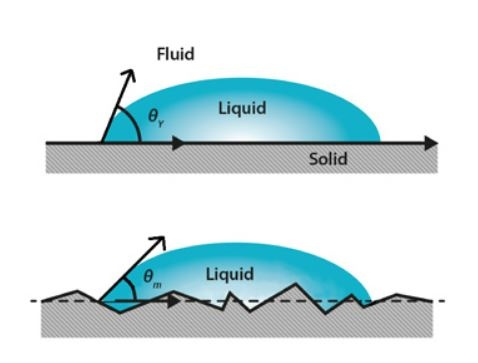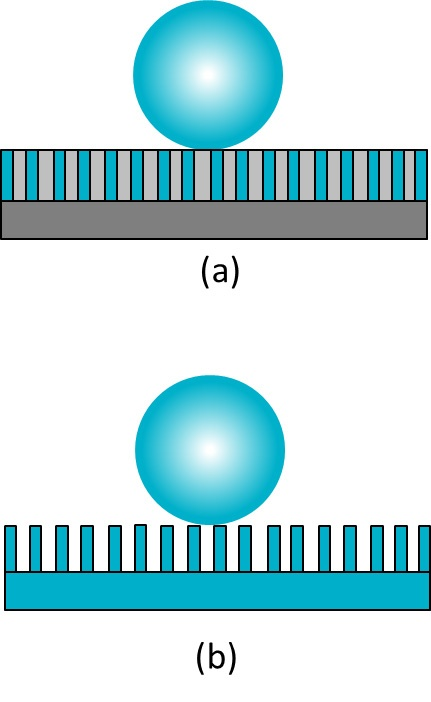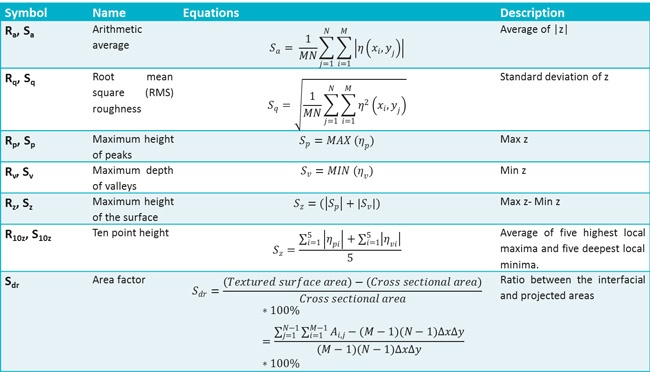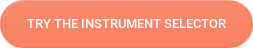Wettability can be studied by measuring the contact angle of a surface with a given liquid. The Young equation that describes contact angle assumes that the surface is chemically homogenous and topographically smooth. This is however not true in the case of most real surfaces. In order to get the actual contact angle, surface roughness and contact angle are to be measured simultaneously to get the roughness corrected contact angle. This can be done with the Attension Theta Topography.

## Contact angle and surface roughness

Both chemical and topographical properties of the surface are important parameters in many different applications and processes, where wetting and adhesion behavior needs to be optimized. Wettability can be studied by measuring the contact angle of the substrate with the given liquid. The well-known Young equation describes the balance at the three-phase contact of solid, liquid and vapor.

γsv = γsl + γlv · cos θY

The interfacial tensions, γsv, γsl and γlv, form the equilibrium contact angle of wetting, often referred to as the Young contact angle θY. The Young equation assumes that the surface is chemically homogenous and topographically smooth. This is however not true in the case of real surfaces, which instead of having one equilibrium contact angle value exhibit a range of contact angles between the advancing and receding ones.

The figure shows the droplet on ideal and real surfaces. On an ideal surface, the Young equation applies and the measured contact angle is equal to the Young contact angle (upper image). On a real surface the actual contact angle is the angle between the tangent to the liquid-vapor interface and the actual, local surface of the solid (lower image). However, the measured (apparent) contact angle is the angle between the tangent to the liquid-vapor interface and the line that represents the apparent solid surface, as seen macroscopically. Actual and apparent contact angle values can deviate substantially from each other. To calculate real surface free energies of the solid the actual contact angles should be used.Contact angles and surface roughness.

The relationship between roughness and wettability was defined in 1936 by Wenzel, who stated that adding surface roughness would enhance the wettability caused by the chemistry of the surface3. For example, if the surface is chemically hydrophobic, it will become even more hydrophobic when surface roughness is added. Wenzel statement can be described with equation below.

cos θm · cos θY

Where θm is the measured contact angle, θY is the Young contact angle and r is the roughness ratio. The roughness ratio is defined as the ratio between the actual and projected solid surface area (= 1 for a smooth surface and > 1 for a rough one) and can be calculated from a 3D roughness parameter Sdr as shown already. It is important to notice that the Wenzel equation is based on the assumption that the liquid penetrates into the roughness grooves (as in Figure 1). It has been stated that if the droplet is larger than the roughness scale by two to three orders of magnitude, the Wenzel equation applies4. Wenzel corrected contact angles have been utilized for example to study the wettability of paper sheets5 and cell adhesion to biomaterial surfaces6. Both micro and nanoscale roughness have been shown to have influence on surface wettability.

For additional information on applications, see:

In cases where the liquid does not penetrate into the grooves, the Wenzel equation does not apply. In this case the Cassie equation is used instead. The Cassie equation was first developed to describe chemically heterogeneous surfaces, with two different chemistries7.

cos θm  x· cos θY1 + x· cos θY2

In the equation above, x is the area fraction characterized by the given chemistry and subscripts 1 and 2 indicate two different surface chemistries (Figure a). If, instead of having different chemistries on the surface, the second area is air like (Figure b), then equation can be written as,

cos θm  x1(cos θY + 1) – 1

Since contact angle against liquid and air can be considered to be 180° (cos θY2 is -1) and the area fraction x2 = 1 – x1. This equation was developed by Cassie and Baxter7 and is thus often called the Cassie-Baxter equation. It has been found that for the droplet to achieve the real Cassie-Baxter stage (no penetration of the liquid inside the grooves), the geometry of the roughness has to be carefully designed8.The most stable contact angle is the one associated with the absolute minimum of the Gibbs energy curve, which can be connected to Young’s contact angle. The contact angles calculated from the Wenzel and Cassie-Baxter equations have been found to be good approximations of the most stable contact angles9.

## Roughness parameters

Surface roughness is a measurement of surface texture. It is defined as a vertical deviation of a real surface from its ideally smooth form. Roughness plays an important role in various processes such as friction and adhesion and is widely measured. Surface roughness cannot be accurately characterized by using a single parameter. Instead, a set of surface roughness parameters is defined. Parameters that characterize surface profiles are called 2D parameters and are marked with the letter ‘R’. These parameters are widely utilized in different applica­tions but are not really able to provide the full information on the three-dimensional surfaces. Parameters to characterize surface topography are called 3D parameters and are marked with the letter ‘S’. Some of the 3D parameters have their 2D counterparts; others are specifically developed for 3D surfaces1. A summary of these parameters as stated by the ISO 25178 (and their 2D counterparts) is presented in table below2, ISO 25178. Sa is an arithmetic mean height of the surface. Sq and its 2D counterpart are the most widely used roughness parameters that give the standard deviation of height. Rp and Rv give the maximum height of the summit and maximum depth of the valleys, respectively. Rz gives the peak-to-peak value and R10z is calculated as a mean height value of five local maxima and local minima. Rz is more sensitive to noise than R10z. The ratio between the interfacial and projected area Sdr gives the additional surface area contributed by the texture. This parameter is especially useful in wettability studies since it can be used to calculate the roughness ratio r, according to equation below.

r = 1 + Sdr ⁄ 100## References

1. K.J. Stout, P.J. Sullivan, W.P. Dong, E. Mainsah, N. Luo, T. Mathia and H. Zahouani, ‘The development of methods for the charac­terization of roughness in three dimensions’ (1993)
2. L. Blunt and X. Jiang, ‘Advanced techniques for the assessment of surface topography, Development of a basis for 3D surface texture standards ‘SURFSTAND’’, Chapter 2, 2003.
[ISO 25178] Geometrical product specifications (GPS) – Surface texture: Areal-Part 2: Terms, definitions and surface texture param­eters.
3. R. N. Wenzel, ‘Resistance of solid surfaces to wetting by water’, Industrial and engineering chemistry 28 (1936) 988.
4. A. Marmur, ‘Soft contact: Measurement and interpretation of contact angles’, Soft Matter 2 (2006) 12.
5. I. Moutinho, M. Figueiredo and P. Ferreira, ‘Evaluating surface energy of laboratory-made paper sheets by contact angle meas­urements’, TAPPI Journal 6 (2007) 26.
6. J. I. Rosales-Leal, M. A. Rodríguez-Valverde, G. Mazzaglia, P. J. Ramón-Torregrosa, L. Díaz-Rodríguez, O. García-Martínez, M. Vallecillo-Capilla, C. Ruiz and M. A. Cabrerizo-Vílchez, ‘Effect of roughness, wettability and morphology of engineered titanium surfaces on osteoblast-like cell adhesion’, Colloids and Surfaces A: Physicochemical and Engineering aspects 365 (2010) 222.
7. A. B. D. Cassie and S. Baxter, ‘Wettability of porous surfaces’, Transactions of the Faraday Society 40 (1944) 546.
8. A. Tuteja, W. Choi, M. Ma, J. M. Mabry, S. A. Mazzella, G. C. Rutledge, G. H. McKinley and R. H. Cohen, ‘Designing superoleo­phobic surfaces’, Science 318 (2007) 1618.
9. A. Marmur, ‘Solid-surface characterization by wetting’, Annual Review of materials research 39 (2009) 473.# Let's find the best tensiometer for your measurements!

Our tensiometer selector guides you to your optimal instrument.